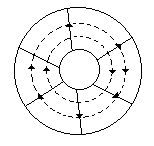# Electronics and Communication Engineering - Microwave Communication

### Exercise :: Microwave Communication - Section 3

16.

In a circular waveguide TE21 mode has lowest cutoff frequency

 A. True B. False

Explanation:

Some applications require dual polarization capability. Circular waveguide has this capability.

These analysis uses cylindrical coordinates.

In circular waveguide TE11 mode has the lowest cut off frequency and is the dominant mode.

If D is diameter of waveguide

λc = 1.706 D for TE11 mode

λc = 1.029 D for TE21 mode

λc = 0.82 D for TE01 mode

λc = 1.306 D for TM01 mode.

17.

The number of TV channels which can be accommodated in a spectrum of 300 MHz is about

 A. 5 B. 10 C. 50 D. 200

Explanation:

A TV channel has a bandwidth about 5 MHz. Hence 300 MHz spectrum can accommodate about 50 channels.

18.

The noise figure of multicavity klystron amplifier is very low

 A. True B. False

Explanation:

A Klystron is a vacuum tube used for generation/amplification of microwaves.

An electron beam is produced by oxide coated indirectly heated cathode and is focussed and accelerated by focussing electrode.

This beam is transmitted through a glass tube. The input cavity where the beam enters the glass tube is called buncher.

As electrons move ahead they see an accelerating field for half cycle and retarding field for the other half cycle.

Therefore, some electrons are accelerated and some are retarded. This process is called velocity modulation.

The velocity modulation causes bunching of electrons. This bunching effect converts velocity modulation into density modulation of beam.

The input is fed at buncher cavity and output is taken at catcher cavity.

In a two cavity klystron only buncher and catcher cavity are used. In multi cavity klystron one or more intermediate cavities are also used.

The features of a multicavity klystron are :

1. Frequency range - 0.25 GHz to 100 GHz

2. Power output - 10 kW to several hundred kW

3. Power gain - 60 dB (nominal value)

A multicavity klystron is used in UHF TV transmitters, Radar transmitter and satellite communication.

19.

Assertion (A): The velocity of electromagnetic waves on overhead lines and coaxial cables is the same.

Reason (R): Free space has an intrinsic impedance of 377 ohms.

 A. Both A and R are correct and R is correct explanation of A B. Both A and R are correct but R is not correct explanation of A C. A is correct but R is wrong D. A is wrong but R is correct

Explanation:

Velocity of em waves on caoxial cables is less than that on overhead lines.

20.

In the given figure the E and H lines in a coaxial cableA. The radial lines are E lines and circular lines are H lines B. Radial lines are H lines and circular lines are E lines C. The directions of E and H lines are wrong D. Radial lines may be E and H lines depending an direction of current